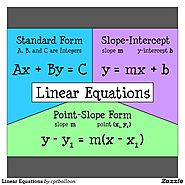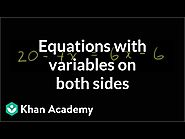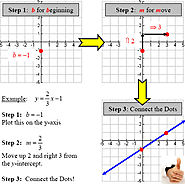Updated by Kayla Steward on May 05, 2019
REPORTKayla Steward
Owner
11 items   1 followers   0 votes   4 views

# T4E: Curated Resources Linear Equations

1

## Equation PracticeDescription: Khan Academy will be used in this instance to practice solving equations algebraically.

Rationale: This website is an excellent refresher for students to practice solving equations algebraically. This material is going to be below grade level but will allow the students to access where they are.

2

## Equation FormsDescription: This image gives the students a clear image of the different forms of linear equations.

Rationale: This picture is simple and brightly colored. The appearance is below grade level, but would provide a good reference point.

3

## Save the ZogsDescription: This game will help students distinguish linear equations on a graph. "Zogs" form a line and the student has to choose the linear equation that matches.

Rationale: I chose this game because it is below grade level and would provide a great intro to the lesson of graphing linear equations.

4

## Linear Functions Word ProblemsDescription: This site provides word problems with multiple choice answers.This will be the "push" exercise more than likely for students.

Rationale: This is on grade level for Algebra 1 students and provides practice with making linear equations to match the problem.

5Description: This game allows for students to solve equations and "shoot" a basket. Rationale: This game will allow for practice on an algebra 1 level and students will get immediate feedback.

6

## Writing linear equations using the slope-intercept form (Algebra 1, Formulating linear equations) – MathplanetDescription: This website is a descriptive tool to walk students through linear equations.

Rationale: I chose this resource because it is on grade level and content related.

7

## Practice Problems & SolutionsDescription: This resource provides six practice problems for students to work through and then check their answers.

Rationale: I chose this because the problems are grade level and provide good practice for algebra 1 students.

8

## Symbolab Math Solver - Step by Step calculatorDescription: Symbolab will be used to check the equations the students are building to see if they calculated them correctly before switching with other students.

Rationale: This site is used to solve equations. Though I want the students to do this on their own, I think it is good for them to check their work and the work of others.

9

## Variables on both sides VideoDescription: This video describes how to solve equations with variables on both sides of the equal sign.

Rationale: This is a great reminder and is a good visual for students, especially those that struggle.

10

## Steps ImageDescription: This image outlines the steps for graphing a linear equation

Rationale: This is a good reference point for students who need step by step directions.

11

## Watch: Slope-Intercept FormSlope-intercept form, y=mx+b, of linear equations, emphasizes the slope and the y-intercept of the line. Watch this video to learn more about it and see some examples.